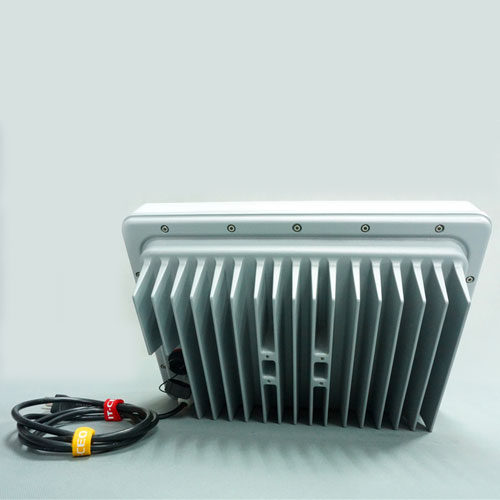• Welcome to jammer-mart device online shop.

# How to test wifi signal strength and choose wifi jammer?

The use of wifi jammer is becoming more and more widespread, but many people do not know how to detect the strength of the signal. The following is an introduction to the method of detecting the strength of the wifi signal jammer.

dBm is a value that evaluates the absolute value of power, and the calculation formula is 10lgP (P=power value/1mw).
[Example 1] If the transmit power P is 1mw, it is 0dBm after converting to dBm.
[Example 2] For a power of 40W, the converted value in dBm should be:
10lg(40W/1mw)=10lg(40000)=10lg4+10lg10+10lg1000=46dBm.

Remember: -70dBm is equal to 10 to the tenth power of a watt, which is one billionth of a watt. Therefore, when using the logarithmic form of dBm to represent extremely small or extremely large power, it is not only very effective but also the original multiplication calculation is converted into a simple addition calculation.
1.4.2 dB
dB is a value that characterizes the relative value. When considering how many dB the power of A is larger or smaller than the power of B, the following formula is used: 10lg (A power / B power)
[Example 3] A's power is twice as large as B's power, then 10lg (A's power/B's power) = 10lg2 = 3dB. That is, A's power is 3 dB greater than B's power.
[Example 4] The 100-meter transmission loss of a 7/8-inch GSM900 feeder is about 3.9dB.
[Example 5] If A's power is 46dBm and B's power is 40dBm, then it can be said that A is 6 dB larger than B.
[Example 6] If antenna A is 12dBd and antenna B is 14dBd, it can be said that A is 2 dB smaller than B.

Base station signal strength and propagation mode

1.5.1 Base Station Signal Strength and Status

Signal Strength Range (dBm) Field Strength Status
-30 or more super high-strength signal area
-30—— -40 Extra strong signal area
-40—— -50 High-strength signal area
-50—— -60 Medium and strong signal area
-60—— -70 Low-strength signal area
-70—— -80 Low and weak signal area
Below -80 High and weak signal area

1.5.2 Base Station Signal Propagation

The effective coverage of a base station is determined by factors such as the transmit power of the base station, the gain of the antenna, and its height angle, which is generally several kilometers. Due to a large number of mobile phones per unit area in the city, the distribution of base stations is relatively dense. Cells in the cell can communicate at the same frequency.

The signal will be attenuated during the propagation. The most basic mechanisms that affect propagation are reflection, diffraction, and scattering.

Reflections occur when electromagnetic waves encounter objects larger than the wavelength, such as the surface of the earth, buildings, and walls.Diffraction occurs when the wireless path between reception and transmission is blocked by a sharp edge, and the secondary waves generated by the blocking surface are dispersed in space, even on the backside of the blocking body. When there is no line-of-sight path between reception and transmission, wave bending also occurs around the barrier.

Scattering occurs when there are objects smaller than the wavelength in the medium through which the electromagnetic wave travels and the number of blocking objects per unit volume are very large, which often occurs on rough surfaces, small objects, or irregular objects. In a practical communication system, leaves, street signs, lamp posts, etc. cause scattering.

Signal propagation generally undergoes the following forms of attenuation:

Free space attenuation: L=32.4 +20logd+20logf (d in kilometers, f in megahertz)

Obstruction attenuation: reinforced concrete structure: ≥28dB

Brick Wall Structure: ≥20dB

Wooden wall, gypsum keel wall: ≥10dB

Anti-theft net: ≥20dB

Glass curtain wall, doors and windows: ≥3dB